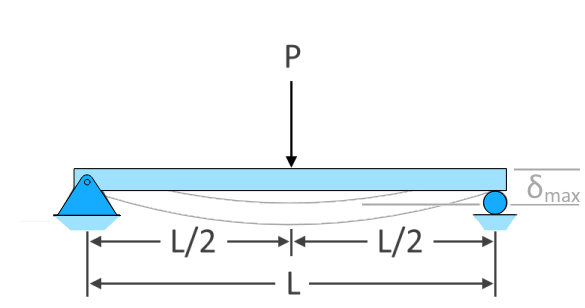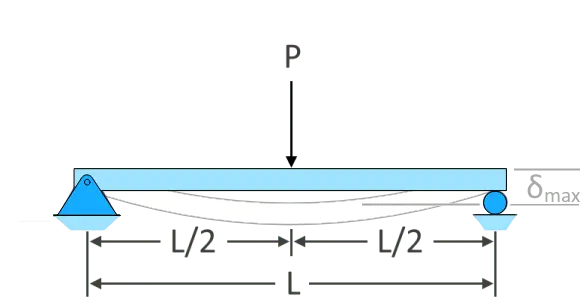Uh Oh! It seems you’re using an Ad blocker!

We always struggled to serve you with the best online calculations, thus, there's a humble request to either disable the AD blocker or go with premium plans to use the AD-Free version for calculators.

Or# Beam Deflection Calculator

Select beam type, load type, and enter the necessary entities. The tool will readily let you know how much deflection is there in the beam.

Beam TypeBeam Type:

Span Length, L:

Modulus of Elasticity, E:Moment of Inertia, Ix:

Distance :

Deflection At:

Table of Content

Get the Widget!

Add this calculator to your site and lets users to perform easy calculations.

Feedback

How easy was it to use our calculator? Did you face any problem, tell us!

Make use of our beam deflection calculator to find maximum deflection of the beam (simple-supported or cantilever) after a certain load is carried on it. Calculates the effect of beam bending depending upon the magnitude and location of the object placed on it.

## How To Calculate Beam Deflection?

Following beam deflection formulas will help you out in determining the respective beam deflections for certain loads it carries:

### Simply-Supported Beam:$$𝛿_{max}=\dfrac{PL^{3}}{48EI}$$$$𝛿_{max}=\dfrac{Pb\left(3L^{2}-4b^{2}\right)}{48EI}$$$$𝛿_{max}=\dfrac{5wL^{4}}{384EI}$$$$𝛿_{max}=\dfrac{0.00652wL^{4}}{EI}$$$$𝛿_{max}=\dfrac{wL^{4}}{120EI}$$

#### Moment Load at Some Support:$$𝛿_{max}=\dfrac{ML^{2}}{9\sqrt{3}EI}$$

### Cantilever Beam:

For these specific types of beam, our steel i beam deflection calculator different equations that are as follows:$$𝛿_{max}=\dfrac{PL^{3}}{3EI}$$$$𝛿_{max}=\dfrac{Pa^{2}\left(3L-a\right)}{6EI}$$

##### Uniformly Varying Load (Case 1):$$𝛿_{max}=\dfrac{wL^{4}}{30EI}$$

##### Uniformly Varying Load (Case 2):$$𝛿_{max}=\dfrac{11wL^{4}}{120EI}$$\(𝛿_{max}=\dfrac{ML^{2}}{2EI})

## How to Use This Beam Deflection Calculator?

To use this beam deflection calculator, follow the below-mentioned steps:

• Select the “Beam Type” and “Load Type.”
• Enter the length of the span and the point load.
• Input the modulus of elasticity and moment of inertia.
• Hit the “calculate” button.

## References:

From the source of Wikipedia: Deflection (engineering), Beam deflection for various loads and supports, Units
From the source of Lumen Learning: Stress, Strain, and Elastic Modulus, Tensile or Compressive Stress, Bulk Stress